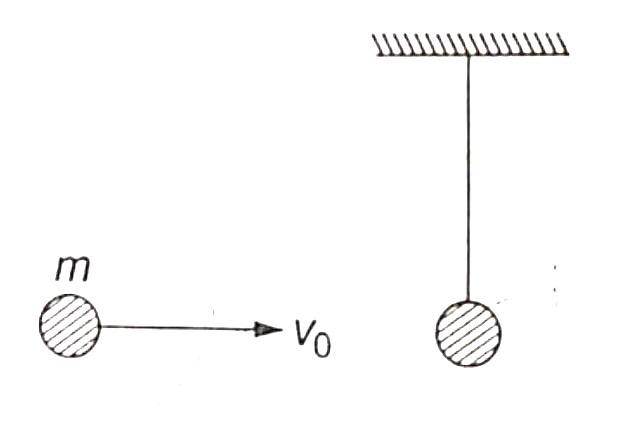You’ve reached the end of your free Videos limit.
#10 | Mindmap
(Physics) > Work,Energy and Power
Unable to watch the video, please try another server

#### My Notes

Create your notes while watching video by clicking on icon in video player.
Related Practice Questions :

If the increase in the kinetic energy of a body is 22%, then the increase in the momentum will be

(1) 22%

(2) 44%

(3) 10%

(4) 300%

High Yielding Test Series + Question Bank - NEET 2020

Difficulty Level:

If momentum is increased by 20%, then K.E. increases by

(1) 44%

(2) 55%

(3) 66%

(4) 77%

High Yielding Test Series + Question Bank - NEET 2020

Difficulty Level:

The kinetic energy of a body of mass 2 kg and momentum of 2 Ns is

(1) 1 J

(2) 2 J

(3) 3 J

(4) 4 J

High Yielding Test Series + Question Bank - NEET 2020

Difficulty Level:

A body of mass ${m}_{1}$ moving with uniform velocity of 40 m/s collides with another mass ${m}_{2}$ at rest and then the two together begin to move with uniform velocity of 30 m/s. The ratio of their masses $\frac{{m}_{1}}{{m}_{2}}$ is

(1) 0.75

(2) 1.33

(3) 3.0

(4) 4.0

High Yielding Test Series + Question Bank - NEET 2020

Difficulty Level:

A sphere of mass m moving horizontally with velocity ${v}_{0}$ collides against a pendulum bob of mass m. If the two masses stick together after the collision, then the maximum height attained is(1) $\frac{{v}_{0}^{2}}{2g}$

(2) $\frac{{v}_{0}^{2}}{4g}$

(3) $\frac{{v}_{0}^{2}}{6g}$

(4) $\frac{{v}_{0}^{2}}{8g}$